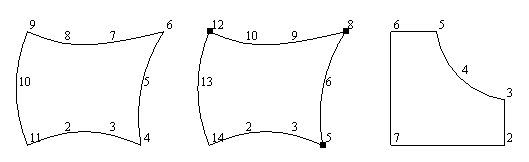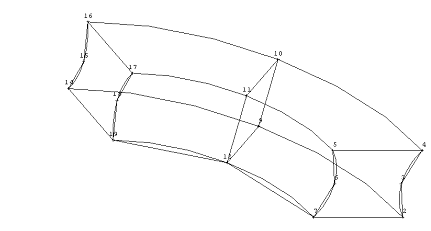# Definition of macro elements within AutoCAD

The macro model should approximate the overall geometrical form of the solid to be calculated by use of large elements („macro elements“). It is important to specify the edges of the solid. The area between the edges is approximated by C0-Coons surfaces. Macro elements are defined by corner nodes and intermediate nodes on edges. For each edge it is possible to specify 10 nodes, for each surface element no more than 20 nodes (40 for type 105 and 400), for each solid element no more than 40 nodes can be specified. Each edge consisting of more than 1 intermediate node between corners will be approximated by a spline curve. Edges with exactly 1 intermediate node will be approximated by a circular arc.

The basis of the element structure will be an AutoCAD generated construction model. For the definition of macro elements it is a good practice to construct additional cross sections of the construction model and to generate additional nodes placed on model edges and intersection edges.

Macro elements can easily be defined following these rules:

Line elements

Line elements are defined with planar or three-dimensional polylines. Polylines having only 2 vertices will be converted to element type 20, not closed polylines with more than 2 vertices are converted to type 25.

Surface elements defined by closed polylines

The easiest way to define surface elements is by defining planar or three-dimensional closed polylines comprising element corner nodes and intermediate nodes.

Two strategies are alternatively used by MAKROS to distinguish between element corner nodes and intermediate nodes on element edges:

1) Succeeding vertices with equal coordinates (denoted as “double vertices”) are used as element corner nodes. Vertices with a small distance (less than 1/50 of the longest distance between two vertices) are also regarded as double vertices.

2) The angles between succeeding edges are checked. Vertices with smallest angles are used as element corner nodes.

The interpretation of a closed polyline got from AutoCAD follows these rules:

First vertex of the polyline defines the first element node.

The polyline is considered to be closed, if the distance between the first and the last vertex is less than 1/50 of the largest distance between two vertices, or if the polyline is marked as closed by AutoCAD.

Polylines with 4 vertices will be converted to type 30 elements; polylines with 5 vertices will be converted to type 40 elements.

Polylines with more than 5 vertices are alternatively converted to type 35 or 45 elements (option T45), to type 105 elements (option T105) or to type 400 elements (option T400), the option must be marked in the corresponding dialog box. With option T400 marked, an element of type 400 is generated with only straight edges; if some edges should be circular, the intermediated nodes on these edges must later be marked with a – sign using command “Element definition”.

To find out the element corner nodes for elements with curved edges, first the presence of double vertices is checked. If double vertices are found, these are interpreted as corner nodes of the element. In case of the presence of a double vertex, all corner nodes of the element must be defined using double vertices. 2 double vertices within the polyline define type 35 elements, 3 double vertices define type 45 elements and more than 3 double vertices define type 105 elements.

If no double vertices can be found within the polyline, the angles between the 2 edges of each vertex are calculated and the vertices with the smallest angles are used as corner nodes. The angles have to be less than 150 degrees (another value can be given within the dialog). If 3 or more vertices with angles less than 150 degrees are found, element type 45 will be generated (where these 3 vertices with the smallest angles are used as corner nodes). With 2 vertices found type 35 elements will be generated. Polylines with more than 3 vertices with angles less than a given value can optionally be stored as type 105 or type 400 elements. This option must explicitly be selected in the dialog.

Between the element corner nodes it is possible to define 0 or more additional nodes on the edges where you can use up to 8 nodes for each edge and up to 20 (40 for type 105 and 400) nodes for the complete element (without double vertices).

Example: In the following figure the left and right elements are defined by a simple polyline where the smallest angles are used to fix the element corners. The second element is defined by a polyline where double vertices are used to fix the element corners. The vertices of the polylines are numbered continuously; a circle marks double vertices. The left polygon consists of 11 vertices, vertices 4, 6, 9 will be used as element corners because these vertices have the smallest angles. The second closed polyline consists of 14 vertices with vertices 4/5, 7/8 and 11/12 with identical coordinates, so these vertices are used as element corners. The right polyline consists of 7 vertices, where the angles in 4 internal vertices are less than 150°; a type 150 element is created because the option T105 is set in the dialog box.Surface elements defined by closed polylines

In case of exactly one intermediate node on each edge type x5 element will be converted  to type x2 element. For exactly 2 intermediate nodes, type x5 will be converted to type x3 element (for FE model only).

For element type 105 and type 400 all vertices must be defined within one plane. We suggest defining these elements as 2D polylines within a local coordinate system.

Several surfaces generated by AutoCAD can be used and directly converted to macro elements. These are the types 3D-surface and 3D-polygon mesh.

3D surfaces will be converted to elements of type 40 or 30.

3D polygon meshes will optionally be converted to type 40, 42 or 45 elements. You can specify, whether all or only some mesh lines are to be used for the generation of the elements. The mesh can be single or double closed.

Solid elements defined by multiple closed polylines

Solid elements are defined by a polyline consisting of at least 2 internal closed polylines. The interpretation of such a polyline generated by AutoCAD is based on the following rules:

Starting from the first vertex the next vertex within a distance of less than eps to the first vertex will be searched (vertex k1, vertex 7 in following figure). If this is the last vertex of the polyline, a surface element will be generated. The polygon area described by these vertices will be interpreted as a surface element of type 3x or 4x (x = 0 or 5). This surface element will become the bottom face of the solid element. The vertex following k1 will be used as the starting vertex for the next closed polygon area and the next vertex within a distance of less than eps to this vertex is searched (vertex k2, vertex 12 in the figure). This area will be considered as a cross section of the solid element (or the top surface of the element, if no further closed polygons follow). Zero or more cross sections between the bottom and top surface can be given.

If the solid element is only built up with straight edges, the fourth and the first vertex and the fifth and the eighth vertex for element type 60, the first and the fifth vertex and the sixth and the tenth vertex for element type 80 are identical. These possibilities are checked first. Type 65 and 85 can consist of up to 8 additional nodes on edges. The total number of nodes must be no more than 40 nodes.

Solid elements can also be defined within AutoCAD as closed 2D polylines with a given object height. This will result in a solid element with constant height where the polyline defines the bottom surface. In case of 4 or 5 polyline vertices, elements of type 6x or 8x are generated. In case of more than 5 vertices, element type 105 is generated with an additional node indicating object height.

Following Fig. defines a solid element by a polyline with 19 vertices (continuously numbered) where vertices 1/7, 8/12 and 13/19 have identical coordinates. With this polygon a solid element of type 82 will be created.Solid element defined by tree closed polylines